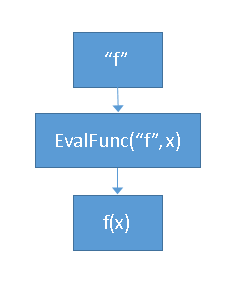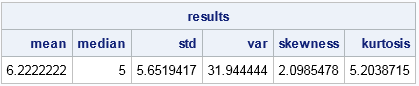A SAS/IML programmer asked whether you can pass the name of a function as an argument to a SAS/IML module and have the module call the function that is passed in. The answer is "yes." The basic idea is to create a string that represents the function call and then use CALL EXECUTE to evaluate the function.

Suppose that you want to create a "super function" (call it EvalFunc) that can compute several univariate statistics such as the mean, the median, the standard deviation, and so forth. Each of these descriptive statistics are calculated by a SAS/IML function that takes one argument. You can therefore define the EvalFunc function to take two arguments: the name of a SAS/IML function and a vector of data. For example, the syntax m = EvalFunc("mean", x) would evaluate mean(x) whereas the syntax s = EvalFunc("std", x) would evaluate std(x). The following SAS/IML function creates a string of the form "z=func(x);" and uses CALL EXECUTE to run that statement:

```proc iml; start EvalFunc(func, x); cmd = "z = " + func + "(x);"; /* z = func(x); */ call execute( cmd ); return z; finish;```

You can test the EvalFunc module by passing the names of some SAS/IML functions that compute descriptive statistics:

```y = {1,2,3,4,5,6,7,8,20}; stats = {"mean" "median" "std" "var" "skewness" "kurtosis"}; results = j(1, ncol(stats), .); do i = 1 to ncol(stats); results[i] = EvalFunc(stats[i], y); end;   print results[colname=stats];```In the example, there is no error checking. It assumes that you pass in a valid function name that takes one argument. Notice that CALL EXECUTE runs immediately in SAS/IML and runs in the local scope. This is in contrast to the way that CALL EXECUTE works in the SAS DATA step. The DATA step pushes statements onto a queue and executes the queue after the DATA step completes.

If you add some IF-THEN/ELSE logic, you could support alternate values of the func argument. For example, if upcase(func)="Q1", you could define cmd = "call qntl(z, x, 0.25);". When you pass that string to CALL EXECUTE, it calls the QNTL function to compute the first quantile (25th percentile).

If you use the SAS/IML Studio application, you can use the ALIAS statement to directly call a function based on the name and signature of that function. The ALIAS function is not supported in PROC IML.

In summary, this article shows that you can pass the name of a function to a SAS/IML module and use CALL EXECUTE to evaluate the function.

Share